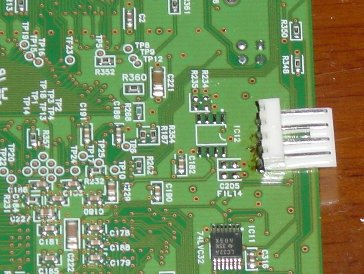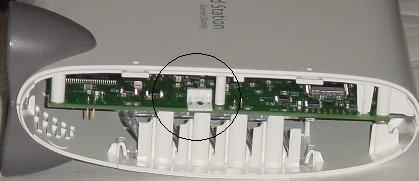# \$B%7%j%"%k%3%s%=!<%k(B

\$B%7%j%"%k%3%s%=!<%k\$r\$D\$1\$F\$/\$l\$H\$\$\$o\$s\$P\$+\$j\$K(B J1 \$B\$H\$\$\$&%Q%?!<%s\$,\$"\$C\$?\$N\$G%3%M%/%?\$r\$D\$1\$F\$_\$?!#(B
LinkStation \$B\$N>l9g(B\$B\$OL\$J1 \$B\$H%7%j%"%k%1!<%V%k\$H\$N7k@~\$O!"(B
 1 \$B%*%l%s%8(B 2 \$B9u(B 3 - 4 \$BCc(B

\$B%7%j%"%k%3%M%/%?\$r(B(USB \$B%7%j%"%k%1!<%V%k7PM3\$G!K(BWindows PC \$B\$K@\B3!"(B
Teraterm Pro \$B\$r5/F0!"(B

 Baud rate 57600bps Data 8 bit Parity none Stop 1 bit Frow control none
\$B\$K@_Dj!"(B
BLR3-TX4 \$B\$K(B AC \$B%"%@%W%?\$+\$i\$N%1!<%V%k\$r;I\$9!#(B